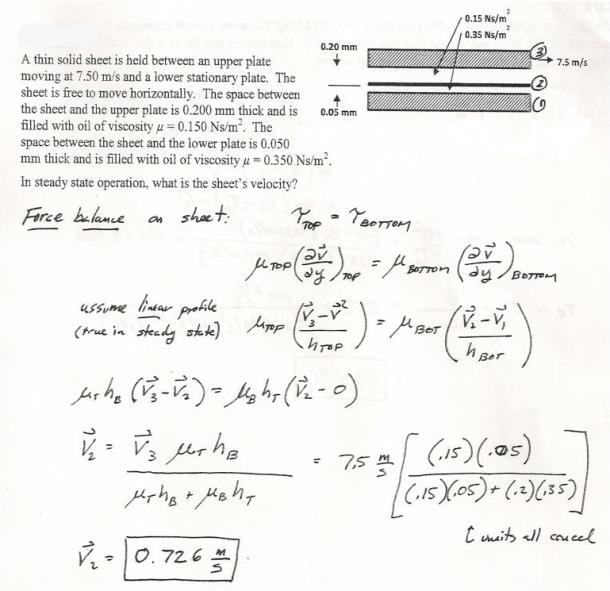fluid mechanics math problems engineering equations fluid mechanics basic mechanics school homework engineering math fluids formulas fluid mechanics problems fluid mechanics problem solutions to fluids problems full solution fluid mechanics engineering fluids problem solution fluid mechanics math problems engineering equations fluid mechanics basic mechanics school homework engineering math fluid mechanics formulas fluid problems fluid mechanics problem solutions to fluid mechanics problems full solution fluid mechanics engineering fluid mechanics problem solution
fluid mechanics math problems engineering equations fluid mechanics basic mechanics school homework engineering math fluids formulas fluid mechanics problems fluid mechanics problem solutions to fluids problems full solution fluid mechanics engineering fluids problem solution fluid mechanics math problems engineering equations fluid mechanics basic mechanics school homework engineering math fluid mechanics formulas fluid problems fluid mechanics problem solutions to fluid mechanics problems full solution fluid mechanics engineering fluid mechanics problem solution
Highalphabet Home Page fluid mechanics solutions fluids math solved Fluid Mechanics Page 1
A thin solid sheet is held between an upper plate moving at 7.5 m/s and a lower stationary plate. The sheet is free to move horizontally. The space between the sheet and the upper plate is .2 mm thick and is filled with oil of viscosity .150 Ns/m^2. the space between the sheet and the lower plate is .05 mm thick and is filled with oil of viscosity .350 Ns/m^2. In steady state operation, what is the sheet's velocity?A thin solid sheet is held between an upper plate moving at 7.5 m/s and a lower stationary plate. The sheet is free to move horizontally. The space between the sheet and the upper plate is .2 mm thick and is filled with oil of viscosity .150 Ns/m^2. the space between the sheet and the lower plate is .05 mm thick and is filled with oil of viscosity .350 Ns/m^2. In steady state operation, what is the sheet's velocity?